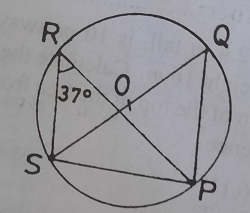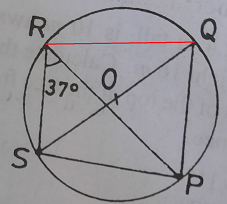mycollegehive
In the diagram, O is the center of the circle. SOQ is the diameter and ∠ SRP = 37o. Find ∠PSQ.
waec-mathematics-2020 math waec-2020 ssce-exam-20200In the diagram, O is the center of the circle. SOQ is the diameter and  ∠ SRP = 37o. Find ∠PSQ.

A. 127

B. 65

C. 53

D. 37

258 viewsShareFollowSchool not set Nigeria
19 August 2020University of Benin Nigeria
19 August 20200∠PQS = ∠SRP = 37

∠RQP = 90

∠RQS = 90 - ∠PQS

= 90 - 37

= 53

∠RQS = ∠PSQ = 53 [Alternate angles]

Ans CShare### Related Tags

waec-mathematics-2020

15 followers

50 questionsmath

8 followers

1015 questionswaec-2020

8 followers

273 questions